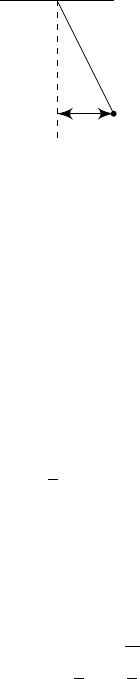2Dimensional analysis

IA Dynamics and Relativity2.2 Scaling
We’ve had so many restrictions on dimensional quantities equations must
be dimensionally consistent, and we cannot sum terms of different dimensions.
However, this is not a hindrance when developing new theories. In fact, it is a
very useful tool. First of all, it allows us to immediately rule out equations that
do not make sense dimensionally. More importantly, it allows us to guess the
form of the equation we want.
Suppose we believe that a physical quantity
Y
depends on 3 other physical
quantities
X
1
, X
2
, X
3
, i.e.
Y
=
Y
(
X
1
, X
2
, X
3
). Let their dimensions be as
follows:
[Y ] = L
a
M
b
T
c
[X
i
] = L
a
i
M
b
i
T
c
i
Suppose further that we know that the relationship is a power law, i.e.
Y = CX
p
1
1
X
p
2
2
X
p
3
3
,
where
C
is a dimensionless constant (i.e. a pure number). Since the dimensions
must work out, we know that
a = p
1
a
1
+ p
2
a
2
+ p
3
a
3
b = p
1
b
1
+ p
2
b
2
+ p
3
b
3
c = p
1
c
1
+ p
2
c
2
+ p
3
c
3
for which there is a unique solution provided that the dimensions of
X
1
, X
2
and
X
3
are independent. So just by using dimensional analysis, we can figure
out the relation between the quantities up to a constant. The constant can
then be found experimentally, which is much easier than finding the form of the
expression experimentally.
However, in reality, the dimensions are not always independent. For example,
we might have two length quantities. More importantly, the situation might
involve more than 3 variables, and we do not have a unique solution.
First consider a simple case if
X
2
1
X
2
is dimensionless, then the relation
between
Y
and
X
i
can involve more complicated terms such as
exp
(
X
2
1
X
2
), since
the argument of exp is now dimensionless.
In general, suppose we have many terms, and the dimensions of
X
i
are not in-
dependent. We order the quantities so that the independent terms [
X
1
]
,
[
X
2
]
,
[
X
3
]
are at the front. For each of the remaining variables, form a dimensionless quan-
tity λ
i
= X
i
X
q
1
1
X
q
2
2
X
q
3
3
. Then the relationship must be of the form
Y = f(λ
4
, λ
5
, ···)X
p
1
1
X
p
2
2
X
p
3
3
.
where f is an arbitrary function of the dimensionless variables.
Formally, this results is described by the Buckingham’s Pi theorem, but we
will not go into details.
Example (Simple pendulum).
m
`
d
We want to find the period P . We know that P could depend on
mass m: [m] = M
length `: [`] = L
gravity g: [g] = LT
2
initial displacement d: [d] = L
and of course [P ] = T.
We observe that
m, `, g
have independent dimensions, and with the fourth,
we can form the dimensionless group
d/`
. So the relationship must be of the
form
P = f
d
l
m
p
1
`
p
2
g
p
3
,
where f is a dimensionless function. For the dimensions to balance,
T = M
p
1
L
p
2
L
p
3
T
2p
3
.
So p
1
= 0, p
2
= p
3
= 1/2. Then
P = f
d
`
s
`
g
.
While we cannot find the exact formula, using dimensional analysis, we know
that if both ` and d are quadrupled, then P will double.